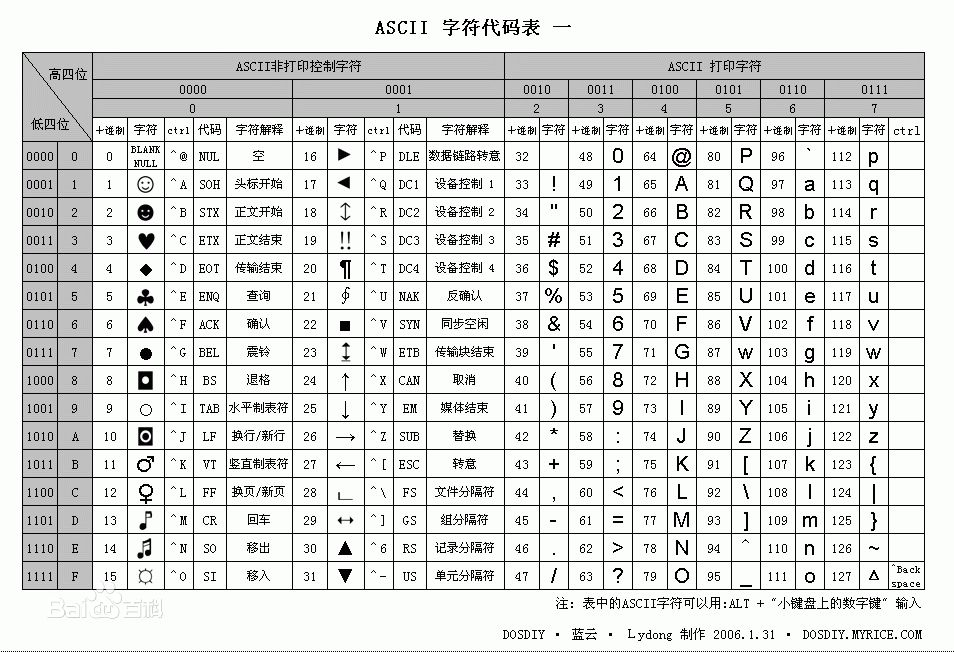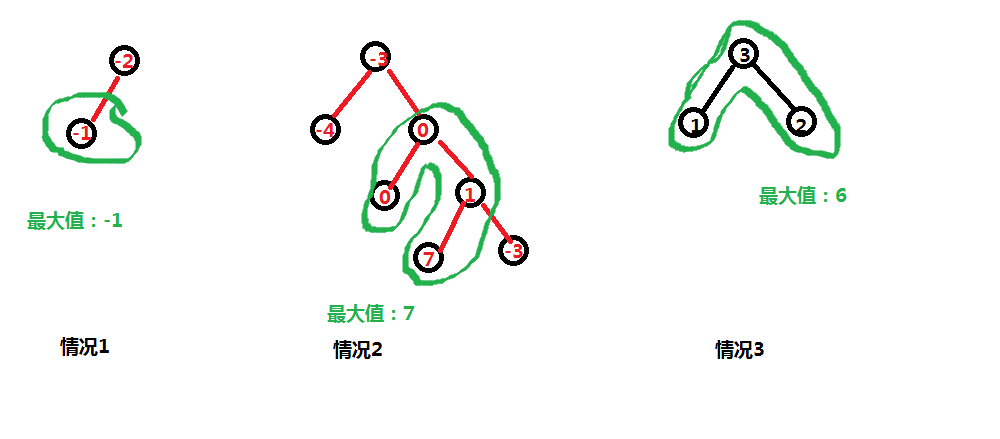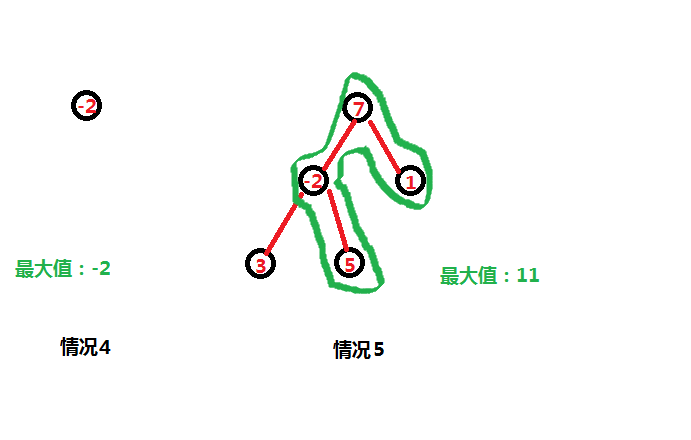# leetCode解题报告5道题(四)

### Longest Consecutive Sequence

Given an unsorted array of integers, find the length of the longest consecutive elements sequence.

For example,
Given [100, 4, 200, 1, 3, 2],
The longest consecutive elements sequence is [1, 2, 3, 4]. Return its length: 4.

Your algorithm should run in O(n) complexity.

Longest Consecutive Sequence

AC代码：

package copylist;

import java.util.HashMap;
import java.util.Map;
/**
*
* @Description:  [求最大连续序列长度]
* @Author:       [胖虎]
* @CreateDate:   [2014-4-5 下午3:12:21]
* @CsdnUrl:      [http://blog.csdn.net/ljphhj]
*/
public class Solution {

Map<Integer,Integer> map = new HashMap<Integer,Integer>();
public int longestConsecutive(int[] num) {
if (num.length == 0)
return 0;
/*初始化Map集合*/
for (int i=0; i<num.length; ++i){
map.put(num[i], 1);
}

return findMaxNum(num);
}
public int findMaxNum(int[] arrays){
int max = Integer.MIN_VALUE;
/*判断数组中的所有元素*/
for (int i=0; i<arrays.length; ++i){
int sum = 0;
int key = arrays[i]; //取出对应的值（即map中的key）

//如果这个key还没有被求解过
if (map.get(key) != -1){
map.put(key, -1);	//置为已求解过
sum++;

/*求左右两侧*/
int left = key - 1;
int right = key + 1;
while (map.containsKey(left) && map.get(left) != -1){
map.put(left, -1);
left = left - 1;
sum++;
}

while (map.containsKey(right) && map.get(right) != -1){
map.put(right, -1);
right = right + 1;
sum++;
}
}
if (sum > max){
max = sum;
}
}

return max;
}
}

### Valid Palindrome

Given a string, determine if it is a palindrome, considering only alphanumeric characters and ignoring cases.

For example,
"A man, a plan, a canal: Panama" is a palindrome.
"race a car" is not a palindrome.

Note:
Have you consider that the string might be empty? This is a good question to ask during an interview.

For the purpose of this problem, we define empty string as valid palindrome.

java中，如果你要判断一个字符是字母或者数字可以用这个函数：Character.isLetterOrDigit(char c)

ASCII码表图：Valid Palindrome

AC代码：

public class Solution {
public boolean isPalindrome(String s) {
int len = s.length();
if (len == 0)
return true;
/*初始化左右两个下标*/
int leftIndex = 0;
int rightIndex = len-1;
/*迭代, 结束的条件是leftIndex < rightIndex 表示整个字符串的字符都访问过了*/
while (rightIndex >= leftIndex){
/*得到当前左右下标各自指向的字符的ASCII码*/
int leftInt = (int)(s.charAt(leftIndex));
int rightInt = (int)(s.charAt(rightIndex));
/*如果左下标指向的字符不属于数字或者字母，下标往后移*/
if (( !(65 <= leftInt && leftInt <= 90)
&& !(97 <= leftInt && leftInt <= 122)
&& !(48 <= leftInt && leftInt <= 57) )){
leftIndex++;
continue;
}
/*如果右下标指向的字符不属于数字或者字母，下标往前移*/
if (( !(65 <= rightInt && rightInt<= 90)
&& !(97 <= rightInt && rightInt<= 122)
&& !(48 <= rightInt && rightInt <= 57) )){
rightIndex--;
continue;
}
/*判断条件，字母的话忽略大小写*/
if( (rightInt == leftInt)
|| Math.abs(rightInt - leftInt) == 32 ){
leftIndex++;
rightIndex--;
}else{
return false;
}
}
return true;
}
}

### Binary Tree Maximum Path Sum

Given a binary tree, find the maximum path sum.

The path may start and end at any node in the tree.

For example:
Given the below binary tree,

       1
/ \
2   3


Return 6.ps:为了方便大家看几种情况数，才画了这么多个情况，实际上1,2,3种情况就够了哈！

1、Null结点对应的值为无穷小（Interget.MIN_VALUE），保证情况4的成立

2、每个子树里面都有可能“左+根+右”的值大于“左+根”或者“右加根”，则我们可以求出这个值（max），代表这个子树所能达到的最大值。这个值再跟现在已经得到的最大值做比较。

3、步骤2已经讲了每个子树都有一个最大值，如果这个最大值满足了最大值路径，那么赋值给当前最大值，否则的话，我们需要得到一个这棵子树过一边的最大值（即“root.val + 左子树的最大值” 或者 “root.val + 右子树的最大值” 或者 "root.val")，因为如果你这棵子树没办法构成最大值路径的话，那么只能由你的上一级树再去做操作了，但是如果你交给上一级树做操作，那么你就不能再同时经过左右两个子树了，你只能选择如果root.val + leftsum > root.val (左+中 > 中) 并且 root.val + leftsum > root.val + rightsum （左+中 > 右）那么你就返回经过“左子树”和“根结点”的这条路径的值给上一级。

Binary Tree Maximum Path Sum

AC代码：

/**
* Definition for binary tree
* public class TreeNode {
*     int val;
*     TreeNode left;
*     TreeNode right;
*     TreeNode(int x) { val = x; }
* }
*/
public class Solution {
/*当前得到的最大值*/
private int maxSum = Integer.MIN_VALUE;

public int maxPathSum(TreeNode root) {
solveSum(root);
return maxSum;
}
/*递归函数*/
public int solveSum(TreeNode root) {
/*如果为Null结点，返回无穷大*/
if (root == null)
return Integer.MIN_VALUE;

int max = 0;

int leftsum = solveSum(root.left);
int rightsum = solveSum(root.right);
int sumLeftRootRight = root.val;

/*下面是为了求出当前这个树中可能的最大路径(可能包括左+中+右)*/
//begin
if (leftsum > 0){
sumLeftRootRight += leftsum;
}
if (rightsum > 0){
sumLeftRootRight += rightsum;
}
//求出这个树中的最大路径值
max = leftsum > rightsum ? leftsum > sumLeftRootRight ? leftsum : sumLeftRootRight : rightsum > sumLeftRootRight ? rightsum : sumLeftRootRight;
//如果这个值大于当前最大值
if (max > maxSum){
maxSum = max;
}
//end

//把经过根结点的一条最大值的路径返回给上一级
//注意:居然要给上一级，那么过这棵子树的根结点的路径的情况
//1. 就一个root结点就最大了
//2. 过了root结点，再过root.left子树的最大路径，最大
//3. 过了root结点，再过root.right子树的最大路径，最大
//passed root node maxsum;
int rootMaxSum = root.val;
rootMaxSum += leftsum > rightsum ? leftsum > 0 ? leftsum : 0 : rightsum > 0 ? rightsum : 0;
return rootMaxSum;
}
}

### Reverse Words in a String

Given an input string, reverse the string word by word.

For example,
Given s = "the sky is blue",
return "blue is sky the".

1、s == null    -->  return null;

2、s == "" || s== " "     -->  return "";

3、s == "   a   b "      --> return "b a";

AC代码：

public class Solution {
public String reverseWords(String s) {

if (s == null || s.equals("")){
return s;
}
StringBuffer buffer = new StringBuffer();
s = s.trim();//取出两边的空格
/*把相邻的空格去除掉*/
for (int i=0; i<s.length(); ++i){
if (s.charAt(i) == ' ' && buffer.toString().charAt(buffer.toString().length()-1) == ' '){
;
}else{
buffer.append(s.charAt(i));
}
}
/*存储逆序的字符串*/
StringBuilder reverseStr = new StringBuilder("");
/*根据空格来做分割*/
String[] arrays = buffer.toString().split(" ");

for (int i=arrays.length-1; i>=0; --i){
if (!reverseStr.toString().equals("")){
reverseStr.append(" ");
}
reverseStr.append(arrays[i]);
}
return reverseStr.toString();
}
}

### Unique Binary Search Trees II

(这个题目的姐妹题：Unique Binary Search Trees I的题解) http://blog.csdn.net/ljphhj/article/details/22616537

Given n, generate all structurally unique BST's (binary search trees) that store values 1...n.

For example,
Given n = 3, your program should return all 5 unique BST's shown below.

   1         3     3      2      1
\       /     /      / \      \
3     2     1      1   3      2
/     /       \                 \
2     1         2                 3


confused what "{1,#,2,3}" means? > read more on how binary tree is serialized on OJ.

AC代码：

package copylist;

import java.util.ArrayList;

class TreeNode {
int val;
TreeNode left;
TreeNode right;

TreeNode(int x) {
val = x;
left = null;
right = null;
}
}
/**
*
* @Description:  [leetcode : Unique Binary Search Trees II ]
* @Author:       [胖虎]
* @CreateDate:   [2014-4-9 上午11:01:15]
* @CsdnUrl:      [http://blog.csdn.net/ljphhj]
*/
public class Solution {

public ArrayList<TreeNode> generateTrees(int n) {
ArrayList<TreeNode> result = new ArrayList<TreeNode>();
if (n == 0){
result.add(null);
return result;
}

result = generateTree(1,n);
return result;
}
/*生成left ~~ right的二叉搜索树的情况*/
public ArrayList<TreeNode> generateTree(int left, int right){
ArrayList<TreeNode> result = new ArrayList<TreeNode>();
if (right < left){
result.add(null);
return result;
}
/*遍历root值分别为 left ~~ right的情况*/
for (int rootvalue=left; rootvalue<=right; ++rootvalue){
/*把左，右子树各自可能的情况分别放在两个集合中.*/
ArrayList<TreeNode> leftResult = generateTree(left, rootvalue-1);
ArrayList<TreeNode> rightResult = generateTree(rootvalue+1, right);
/*两个集合的组合数为最后的结果*/
for (int j=0; j<leftResult.size(); ++j){
for (int k=0; k<rightResult.size(); ++k){
TreeNode rootNode = new TreeNode(rootvalue);
rootNode.left = leftResult.get(j);
rootNode.right = rightResult.get(k);
result.add(rootNode);
}
}
}
return result;
}
public static void main(String[] args) {
Solution s = new Solution();
ArrayList<TreeNode> list = s.generateTrees(4);
}
}03-02
10-152104
04-023987
04-033159
09-02319
03-272万+
10-25711
07-12
05-0671
09-066万+
07-041万+
10-18851
12-0621万+
04-102559
04-13570
06-233962
©️2020 CSDN 皮肤主题: 酷酷鲨 设计师:CSDN官方博客